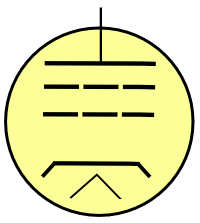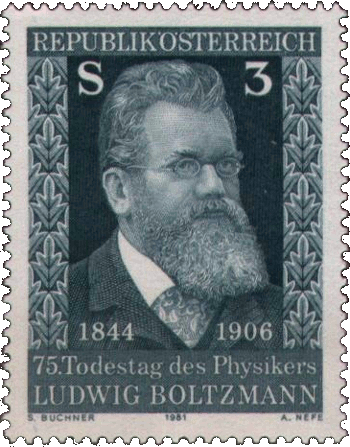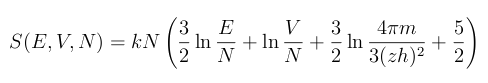### Sackur-Tetrode

February 2, 2012

Whenever I hear the word, "tetrode," I think of the four-element vacuum tube by that name. This tube has four elements (tetra-, τετρα-, is the Greek prefix for the number four), but only if you ignore the filament that's required to bring the cathode to the temperature required for thermionic emission of electrons. Someone who's a little younger, and more physicist than electrical engineer, would recall the Sackur-Tetrode equation. The hundredth anniversary of the Sackur-Tetrode equation is this year.Vacuum tube nostalgia - A tetrode.

The elements, from top to bottom, are the plate, screen grid, control grid, cathode and filament.

Illustration by the author, rendered with Inkscape)

Otto Sackur (1880-1914) was a German physical chemist who is known principally for this eponymous equation. Hugo Tetrode (1895-1931) was a Dutch theoretical physicist who hobnobbed with such luminaries as Einstein, Paul Ehrenfest and Hendrik Lorentz. He published many papers on quantum mechanics in Zeitschrift für Physik, and other German physics journals.

Tetrode was somewhat of a prodigy, having published his first paper at age seventeen in the Annalen der Physik, the preeminent physics journal of the era and the vehicle for Einstein's important papers. Like Paul Dirac, he didn't interact well with people. He even refused a visit from Einstein and Ehrenfest when they called at his Amsterdam residence. Tetrode died, unmarried, at the early age of thirty-five.

The Sackur-Tetrode equation wasn't a collaborative effort of Sackur and Tetrode. They developed the equation independently of each other in 1912. The equation was the first application of quantization to particle states. Until that time, only photons were considered as quanta, with their energy E specified by Planck's famous equation, E = hν, where h is Planck's constant and ν is frequency.

The quantization of photon energy handily solved some nagging problems in electromagnetism, some of which were given interesting names, like the "ultraviolet catastrophe." There was a similarly nagging problem in thermodynamics involving entropy. Boltzmann's entropy formula, S = k ln Ω, specifies the entropy S as a function of the number of possible states of a system, Ω. This leads to a problem of enumerating the number of possible states of gas molecules in a volume.Ludwig Boltzmann, as depicted on a 1981 Austrian stamp.

Boltzmann was a modern proponent of atomism at a time when many physicists doubted the existence of atoms. Boltzmann argued that much good physics could be done if atoms were merely supposed to exist, so no one should object to his statistical mechanics simply because of their views on atomism.

(Via Wikimedia Commons))

If phase space were continuous, a molecule would have an infinite space to explore. Sackur and Tetrode reasoned that the volume of phase space for a gas must be hn, where n is the number of degrees of freedom. In doing so, they applied quantum theory to a statistical mechanics problem fourteen years before the 1926 Schrödinger equation. Tetrode's exposition is easier to follow than Sackur's, possibly because we've been schooled along the lines of his quantum mechanical reasoning.

Getting the math to work, and convincing others that your equation is an actual representation of reality, are two different things. Walter Grimus, a physicist at the University of Vienna, and author of a paper on the equation in recognition of its hundredth anniversary, writes that it was important that both Sackur and Tetrode showed the concurrence of their equation with experimental data. They used thermodynamic data for mercury, the vapor of which is monoatomic and a good approximation to an ideal gas.

To make the connection to entropy, Sackur and Tetrode needed quite a bit of data. They needed as a function of temperature the vapor pressure of mercury, the latent heat of vaporization, and heat capacity of its solid phase. Fortunately, such data were available in the published literature. In his paper, Grimus shows that a calculation of Planck's constant using the equation and modern values of the mercury data gives a result accurate to a percent.

As for the equation itself, I'm leaving it in a footnote. It's too complicated to explain in such a short article, so I'm following the advice of Stephen Hawking's editor for his first popular book, A Brief History of Time, that every equation halves the number of readers. Hawking did include one equation in this book, E = mc2, which is as much a cultural icon as an equation.

Sackur is known principally for this equation, since he died at the young age of 34, just two years after its publication. Sackur died in an explosion in Fritz Haber's laboratory.

### References:

1. W. Grimus, "On the 100th anniversary of the Sackur-Tetrode equation," arXiv Preprint Server, December 16, 2011.
2. The Sackur-Tetrode equation (Eq. 9 of Ref. 1, via the arXiv Preprint Server)Linked Keywords: Tetrode; vacuum tube; Greek; filament; cathode; temperature; thermionic emission; electron; physicist; electrical engineer; Sackur-Tetrode equation; plate; screen grid; control grid; ; Inkscape; Otto Sackur; German; physical chemist; Hugo Tetrode; Dutch; theoretical physicist; Einstein; Paul Ehrenfest; Hendrik Lorentz; quantum mechanics; Zeitschrift für Physik; journal; prodigy; Annalen der Physik; Paul Dirac; Amsterdam; photon; quantum; energy; Planck's famous equation; Planck's constant; frequency; electromagnetism; ultraviolet catastrophe; thermodynamics; entropy; Ludwig Boltzmann; entropy formula; gas molecule; volume; Austrian; atomism; physicist; physics; statistical mechanics; Wikimedia Commons; phase space; infinite; degrees of freedom; Schrödinger equation; Walter Grimus; University of Vienna; experiment; mercury; vapor; monoatomic; ideal gas; vapor pressure; latent heat of vaporization; heat capacity; solid phase; Stephen Hawking; A Brief History of Time; Fritz Haber; laboratory; arXiv Preprint Server.